# Intermediate NumPy¶

### Modules - Basics¶

Last edited: November 21th 2019

In this intermediate NumPy notebook we go through some of the more common functions and features of NumPy. It is intended as a continuation of our notebook on the very basics of NumPy, found here. The notebook is somewhat lengthy. The different sections are intended to be readable on their own, so feel free to jump to the section that you are interested in.

In :
import numpy as np
import matplotlib.pyplot as plt


## Arrays of different shapes¶

Arrays can have any dimension and shape that we wish. This makes them very powerful. Let us transform $$(0, 1, 2, 3, 4, 5, 6, 7, 8)$$ into $$\begin{bmatrix} 0 & 1 & 2\\ 3 & 4 & 5\\ 6 & 7 & 8 \end{bmatrix}.$$

In :
# Create a 3x3 matrix

A = np.arange(9)  # Points from 0 through 8, with spacing 1
print("A before reshape:", A)
A = A.reshape((3,3))  # Set shape to 3x3
print("A after reshape:")
print(A)

A before reshape: [0 1 2 3 4 5 6 7 8]
A after reshape:
[[0 1 2]
[3 4 5]
[6 7 8]]

In :
# We can also get an empty array with given size directly from np.zeros
np.zeros((4, 2))

Out:
array([[0., 0.],
[0., 0.],
[0., 0.],
[0., 0.]])

Two dimensional arrays can be used just like matrices, which makes it easy to express our mathematical expressions in code. In NumPy, the symbol for matrix or vector multiplication is @.

In :
x = np.array([0, 1, 2])
print(A @ x)  # Print the result of A matrix multiplied with x

[ 5 14 23]


Read more about linear algebra in NumPy in this notebook, which covers solving system of equations, eigenvalue problems and more.

Having matrix-shaped arrays becomes especially useful once we have learned slicing.

## Slicing and indexing¶

When we have arrays, be it one or multi-dimensional, it is useful to be able to refer to only the elements we want, just like we do with indexing in normal lists.

Lets review normal lists first. Remember that lists are 0-indexed, that is the first element is element 0, the second is 1 and so on.

In :
my_list = [1,2,3,4]  # Normal Python list

# We can access different parts of the list by slicing and indexing:
print("my_list:\t", my_list)     # First element
print("my_list[:2]:\t", my_list[:2])   # The two first elements
print("my_list[-2:]:\t", my_list[-2:]) # The last two elements

my_list:	 1
my_list[:2]:	 [1, 2]
my_list[-2:]:	 [3, 4]


In general the syntax for slicing a lists is my_list[start:end:step], where start is the first element we want, end is the last element (non-inclusive), and step is the step size. Note that having start or end empty, will slice from the start or to the end, respectively. Negative values count from the end, so that -1 is the last element.

If you are completely unfamiliar with this, you are advised to play around with it now, before moving on to array slicing.

We can do the same with arrays, but arrays have even more ways of slicing!

The syntax for slicing in NumPy is exactly the same as for lists, but we can do it for each dimension! For a two-dimensional array the syntax then becomes my_array[start_1:end_1, start_2:end_2], where start_1 and end_1 is the start and end values for the first axis, and similarly with start_2 and end_2 for the second axis.

Let us again consider the matrix $$A = \begin{bmatrix} 0 & 1 & 2\\ 3 & 4 & 5\\ 6 & 7 & 8 \end{bmatrix}$$ represented as an array.

In :
# Generate the array
# A = [0, 1, 2]
#     [3, 4, 5]
#     [6, 7, 8]
A = np.arange(9).reshape((3,3))

In :
# Let us access some elements

# A_00, first row, first column (remember 0-index, in mathematical notation this would be A_11)
print("A_00:", A[0,0])

# A_02, first row, third column
print("A_02:", A[0,2])

# A_21, third row, last second column
print("A_21:", A[2,1])

A_00: 0
A_02: 2
A_21: 7

In :
# We can go even more advanced, and get entire columns and rows

# First row of A
print("First row:", A)

# First column of A
print("First column:", A[:,0])

First row: [0 1 2]
First column: [0 3 6]


Getting the first row is something we can do with plain old nested python lists, getting the first column, however, would require a for-loop with lists. With arrays this is achieved with a simple index, as shown in the example. At first this might seem very confusing, but we will break it down to make it understandable.

Remember from our list-review that : means all elements, since both start and end is empty. For example my_list[:] just gives us my_list. In A[:, 0] we mean "entire list in first axis, 0th element of second axis", that is any row, first column. Building on this notation, we remember that my_list[1:] gives us the entire list starting from the second, index 1, item. We can use this in arrays to get parts of a column or row.

In :
# First column of A, starting from second element in column
print("A[1:, 0]:", A[1:, 0])

# We can of course also get other columns
print("A[1:, 2]:", A[1:, 2])

A[1:, 0]: [3 6]
A[1:, 2]: [5 8]


Try to convince yourself as to why the following line gives us the result that it does:

In :
print("A[1:, 1:]:")
print(A[1:, 1:])

A[1:, 1:]:
[[4 5]
[7 8]]


## Meshgrid¶

Meshgrid may appear confusing at first, but it is very useful and once you get the grasp of it, you will hopefully realize that it is quite simple. Before explaining what meshgrids are, let us explain why we need them. Imagine that you were to plot a heatmap, that is a 2D plot where each point on the plane is given a color dependent on the value of the function that you are plotting. Recall that when plotting in matplotlib, which you can read more about here, one has to supply two lists, one with $x$-values and one with $y$-values. This gives a set of points, where the function will be plotted.

When plotting in the plane, one needs three lists of values, the $x$ and $y$ coordinates, and the function values at each point. For each $x$ value, we want to iterate over all the $y$ values, so that every point is covered. If we just passed our $x$- and $y$-arrays directly, however, we would get only one point for each $x$-value, and likewise for the $y$-values. This is where meshgrid comes into play. For the two dimensional case, it will give us two arrays, where each value is repeated in such a way that the two lists forms a grid. This is better demonstrated by an example.

In :
x = np.arange(0, 5)
y = np.arange(2, 5)

xx, yy = np.meshgrid(x, y)
print('x =',x, ', y =', y, '\n')
print(xx, '\n')
print(yy)

x = [0 1 2 3 4] , y = [2 3 4]

[[0 1 2 3 4]
[0 1 2 3 4]
[0 1 2 3 4]]

[[2 2 2 2 2]
[3 3 3 3 3]
[4 4 4 4 4]]


Notice that if you were to extract corresponding elements in xx and yy, i.e. the elements with the same position in the matrices, you would get pairs of $x$ and $y$ values. We have thus assigned coordinates to each point, just like we wanted! It is also worth noting that xx and yy have the same shape. We can now plot our result.

In :
plt.pcolormesh(xx, yy, xx + yy)  # pcolormesh plots a heatmap
plt.show()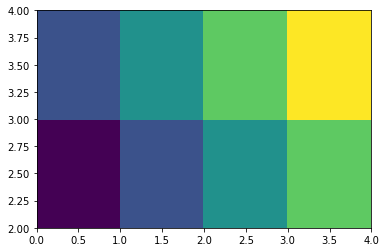Computation speed considerations:

Meshgrid is not only useful for plotting. It enables us to do calculate values over multiple values very easily. Remember that with NumPy we can calculate a value over an array by simply passing the array to the function. We can do this with meshgrids as well, they are nothing more than two dimensional arrays. This helps us avoid nested for-loops, which greatly speeds up our computations and makes the code easier to write and read.

Mehsgrids are useful for all functions that are functions of two variables. As an example, we will look at the steady state amplitude of a driven damped harmonic oscillator which is an expression derived in classical mechanics. It is given by $$\Theta_0(q, \omega_D) = \frac{F_D}{(\omega_0^2 + \omega_D^2)^2 + (q\omega_D)^2},$$ where $F_D$ is the driving force, $\omega_0$ is the eigenfrequency of the oscillator, $q$ is the damping factor, and $\omega_D$ is the driving frequency.

We want to look at this amplitude for different $q$ and $\omega_D$.

In :
def amplitude(omega, omega_drive, q):
"""Amplitude of a driven harmonic oscillator
with eigenfrequency omega, external force with
frequency given by omega_drive, and a damping
constant q. Here the driving force F_D is equal to 1."""

return 1/np.sqrt((omega**2-omega_drive**2)**2 + (q*omega_drive)**2)

omega = 1
q = np.linspace(0.5, 1.5, 20)
omega_D = omega * np.linspace(0.5, 1.5, 40)

qq, omega_DD = np.meshgrid(q, omega_D)

In :
# Calculate the amplitude for each omega_D and q
a = amplitude(omega, omega_DD, qq)
# If X and/or Y are 1-D arrays they will be expanded
# as needed into the appropriate 2-D arrays.
# I.e. no need of np.meshgrid in this case.
plt.pcolormesh(omega_D, q, a.T)  # a.T returns the transpose of a
plt.colorbar(label='Amplitude')
plt.ylabel("q")
plt.xlabel("$\omega_D$")
plt.show()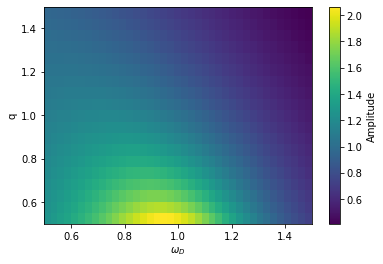We will now investigate the calculation times further. To do this, we will use Jupyter's %timeit function. It calculates the time it takes to execute a command or cell. Lines prefixed by %timeit will be timed on their own. Starting a cell with %%timeit, will time the whole cell.

In :
%timeit a = amplitude(omega, omega_DD, qq)

8.97 µs ± 99.3 ns per loop (mean ± std. dev. of 7 runs, 100000 loops each)

In :
%%timeit
# Equivalent calulation, using for-loops.
a_python = []
for oD in omega_D:
for q_val in q:
a_python.append(amplitude(omega, oD, q_val))

2.22 ms ± 71.9 µs per loop (mean ± std. dev. of 7 runs, 100 loops each)


With computation times of 9.0$\mu$s and 2.2ms, it is obvious that the difference in computation time is significant! Computational speed will be of great importance as the size of the data becomes big.

In :
# Greatly increase the number of points from previous example!
omega = 1
q = np.linspace(0.5, 1.5, 2000)
omega_D = omega * np.linspace(0.5, 1.5, 4000)

qq, omega_DD = np.meshgrid(q, omega_D)
%timeit a = amplitude(omega, omega_DD, qq)

73.8 ms ± 14.7 ms per loop (mean ± std. dev. of 7 runs, 10 loops each)

In :
%%timeit
a_python = []
for oD in omega_D:
for q_val in q:
a_python.append(amplitude(omega, oD, q_val))

24 s ± 2.28 s per loop (mean ± std. dev. of 7 runs, 1 loop each)


For the curious reader, we will mention that this could also have been achieved using NumPy's broadcasting functionality. It is a more direct method, but less intuitive.

## Complex numbers¶

NumPy has built in support for complex numbers. They are intuitive to work with, and makes it easy to put mathematics into code.

NumPy uses j for the imaginary unit. Let us see it in action.

In :
print(1j**2)  # Should give -1

(-1+0j)


To make NumPy understand that we wish to work with complex numbers, we must explicitly set the imaginary part, even when it is zero.

In :
# Fails
print(np.sqrt(-1))

# Works
print(np.sqrt(-1+0j))

nan
1j

/usr/lib/python3.7/site-packages/ipykernel_launcher.py:2: RuntimeWarning: invalid value encountered in sqrt


In :
# Array with imaginary numbers between 0 and 2pi j
a = np.linspace(0+0j, 2*np.pi*1j, 50)

In :
plt.plot(np.abs(a), np.real(np.exp(a)), label=r'Re$[e^{ia}]$')  # Plot real value of np.exp(a)
plt.plot(np.abs(a), np.imag(np.exp(a)), label=r'Im$[e^{ia}]$')  # Plot imaginary value of np.exp(a)
plt.legend()
plt.xlabel('$a$')
plt.show()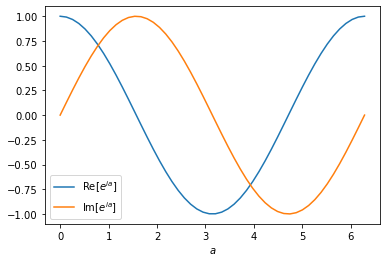We observe a $90^{\circ}$ phase difference between the real and imaginary part, just like expected.

## Reading and writing to file¶

Using only built-in Python functions for reading from and writing to files can be tedious. NumPy has functions designed specifically for reading and writing structured data, np.loadtxt and np.savetxt. The functions are very powerful, and have lots of options. We will here focus on the basic usage, and refer the reader to the NumPy documentation for more advanced usage.

The data file used in this example can be found here.

loadtxt will read the data in the file, convert it to an appropriate data type, and store it in a NumPy array. Let us see it in action.

In :
# From the header line in my_data.txt,
# we know that the file is in the format
# time  temperature  wind
print(data)

[[ 0.         10.52220137  3.15239917]
[ 0.52631579 10.23355002  3.27285991]
[ 1.05263158 10.92881718  3.53663193]
[ 1.57894737 10.4880909   3.50229028]
[ 2.10526316 10.43978719  3.47322148]
[ 2.63157895 10.40069219  4.58370474]
[ 3.15789474 10.38539     4.92386551]
[ 3.68421053 10.82980119  4.74906494]
[ 4.21052632 10.45174438  5.55397891]
[ 4.73684211 10.16078747  6.14630413]
[ 5.26315789 10.10223829  6.07077798]
[ 5.78947368 10.59938701  7.21440152]
[ 6.31578947 10.97894173  7.69948852]
[ 6.84210526 10.63288969  9.74896976]
[ 7.36842105 10.48996214  9.39695595]
[ 7.89473684 10.53800563 11.24194734]
[ 8.42105263 10.36691713 11.21430356]
[ 8.94736842 10.51385622 12.03454861]
[ 9.47368421 10.04225154 12.11379482]
[10.         10.64815814 13.05222008]]


Notice that each row in our array corresponds to a time. Often, it would be more convenient to have each data series in its own array, for example if we wanted to plot our data. We could achieve this with NumPy slicing, but loadtxt has a more direct way of doing this.

In :
t, temperature, wind = np.loadtxt('my_data.txt', unpack=True)


unpack=True transposes our data, so that each row represents one field, in this example time, temperature, and wind. This way, we can easily unpack the data into our variables t, temperature, and wind.

In :
plt.plot(t, temperature, 'o-', label="Temperature")
plt.plot(t, wind, 'o-', label="Wind")
plt.legend();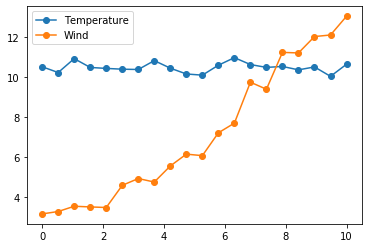We will now generate some new data, that we can store to a file, my_new_data.txt.

In :
# Mean of temperature
T_mean = np.mean(temperature)
# Deviation from mean at each time
delta_temperature = temperature - T_mean
# Save to file
np.savetxt('my_new_data.txt', np.transpose((t, delta_temperature)))


A lot happen in the final line, so we will analyze it further here. savetxt takes the data to be written to file as second argument, in this example it is np.transpose((t, delta_temperature)). Why the transpose, one might ask; we wish to have t and delta_temperature as columns, not rows, so that it has the same format as our input file.

## Useful NumPy functions and examples of usage¶

In this section you will find some useful functions with a short explanation. For a more detailed explanation, please visit the NumPy documentation. After the list of functions follow some examples of using NumPy to solve various problems one might encounter. This is intended as an inspiration to begin thinking in the "NumPy mindset".

Some useful functions:

• np.amin, np.amax. Returns min and max value of array.

• np.argmin, np.argmax. Returns the index of the min and max value of an array.

• np.argwhere. Returns the indices where a condition is true. For example
a = np.array([0,1,2,3,10])
np.argwhere(a>1)
would yield [2,3,4].

### Coefficients of wave function¶

The physics of this example is not important for understanding NumPy. If you do not understand this, do not worry!

We know from linear algebra that given a orthonormal basis $B$ of $V$, any vector $v\in V$ can be written as a linear combination of vectors $b \in B$ . We also know that the coefficient of $b\in B$ for some $v\in V$ is given by $$c_b = \langle b, v\rangle.$$

This is used much in physics, probably most noteworthy in quantum mechanics. Here follows a quick example of using NumPy for linear algebra, in order to calculate the coefficients of for different wave functions.

In :
x = np.linspace(0, 1, 5000)
dx = x - x

# Basis wave functions for particle in a box potential
# These functions can be easliy derived using basic quantum mechanics
g1 = lambda x: np.sqrt(2)*np.sin(np.pi*x)
g2 = lambda x: np.sqrt(2)*np.sin(2*np.pi*x)
g3 = lambda x: np.sqrt(2)*np.sin(3*np.pi*x)

# Create some linear combinations
y1 = g1(x) + g2(x)
y2 = g1(x) + 2*g2(x)
y3 = 0.2*g1(x) + 10*g3(x)

# Stack the functions vertically, creating matricies
basis_functions = np.vstack((g1(x), g2(x), g3(x)))
my_functions = np.vstack((y1, y2, y3))

# Take the matrix product
basis_functions*dx @ my_functions.T

Out:
array([[1.00000000e+00, 1.00000000e+00, 2.00000000e-01],
[1.00000000e+00, 2.00000000e+00, 1.35873630e-15],
[1.69804390e-15, 1.41392499e-15, 1.00000000e+01]])

We can now read the coefficients of each function y1, y2, and y3 from the columns. For example, first column shows that y1 has coefficients 1, 1, and $1.7\cdot 10^{-15}\approx 0$, as expected.

### Find minima of function¶

Consider the function $$f(x) = -\frac{1}{6}x^3 + 2x.$$

Find its local minima.

In :
x = np.linspace(-5, 5, 50)

def f(x):
return -x**3/6 + 2*x
plt.plot(x, f(x))
plt.show()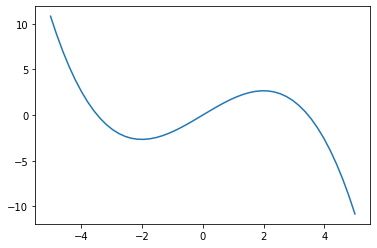We see that the local minima is left of 0, and that there are smaller values than this minima right of zero. It is therefore smart to limit our list of $x$'s to those that are negative.

x[x<0]

gives us exactly that. Now we just pass this array to f, and find its minima using np.min.

In :
np.min(f(x[x<0]))
y_min = np.min(f(x[x<0]))


This gives us the $y$-value at the local minima. We also wish to find the corresponding $x$-value. np.argmin gives us the index of the $y$-value with the minimal value, using this index on the $x$-array gives us the corresonding value.

In :
min_arg = np.argmin(f(x[x<0]))
x_min = x[x<0][min_arg]
print(f"(x,y) = {x_min:.2f}, {y_min:.2f}")

(x,y) = -1.94, -2.66


#### Some general considerations regarding computation speed¶

The main advantage of using NumPy in numerical computations, in addition to adding a simple and intuitive way to write our code, is the significantly increased computation speed. Here are some points worth remembering, in order to best take advantage of this.

Use NumPy arrays! The most important step is to actually use NumPy arrays. With a few exceptions, all lists that one uses, should be NumPy arrays.

Use the NumPy functionality. Take use of the NumPy arrays. Inexperienced NumPy users have a tendency to solve their problems using more conventional Pythonic solutions, when it would be advantageous to use NumPy's functionality. For example, one should very seldom need to use for-loops, as these can often be substituted with NumPy operations, which are much faster.

Do not append to NumPy arrays. One should try to avoid appending to NumPy arrays. It is significantly slower than initializing your array first, and then writing to it. A good example is iterative methods with a fixed number of iterations, like Euler's method. Initializing the $y$-array first with either zeros (np.zeros) or empty values (np.empty), is faster than appending for each time step.